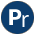#pairs.pl -- Operations on key-value lists

This module implements common operations on Key-Value lists, also known as Pairs. Pairs have great practical value, especially due to keysort/2 and the library assoc.pl.

This library is based on discussion in the SWI-Prolog mailinglist, including specifications from Quintus and a library proposal by Richard O'Keefe.

author
- Jan Wielemaker
- keysort/2, library(assoc)
pairs_keys_values(?Pairs, ?Keys, ?Values) is det
True if Keys holds the keys of Pairs and Values the values.

Deterministic if any argument is instantiated to a finite list and the others are either free or finite lists. All three lists are in the same order.

- pairs_values/2 and pairs_keys/2.
pairs_values(+Pairs, -Values) is det
Remove the keys from a list of Key-Value pairs. Same as `pairs_keys_values(Pairs, _, Values)`
pairs_keys(+Pairs, -Keys) is det
Remove the values from a list of Key-Value pairs. Same as `pairs_keys_values(Pairs, Keys, _)`
group_pairs_by_key(+Pairs, -Joined:list(Key-Values)) is det
Group values with equivalent (==/2) consecutive keys. For example:
```?- group_pairs_by_key([a-2, a-1, b-4, a-3], X).

X = [a-[2,1], b-, a-]```

Sorting the list of pairs before grouping can be used to group all values associated with a key. For example, finding all values associated with the largest key:

```?- sort(1, @>=, [a-1, b-2, c-3, a-4, a-5, c-6], Ps),
group_pairs_by_key(Ps, [K-Vs|_]).
K = c,
Vs = [3, 6].```

In this example, sorting by key only (first argument of sort/4 is 1) ensures that the order of the values in the original list of pairs is maintained.

Arguments:
 Pairs - Key-Value list Joined - List of Key-Group, where Group is the list of Values associated with equivalent consecutive Keys in the same order as they appear in Pairs.
transpose_pairs(+Pairs, -Transposed) is det
Swap Key-Value to Value-Key. The resulting list is sorted using keysort/2 on the new key.
map_list_to_pairs(:Function, +List, -Keyed)
Create a Key-Value list by mapping each element of List. For example, if we have a list of lists we can create a list of Length-List using
`        map_list_to_pairs(length, ListOfLists, Pairs),`